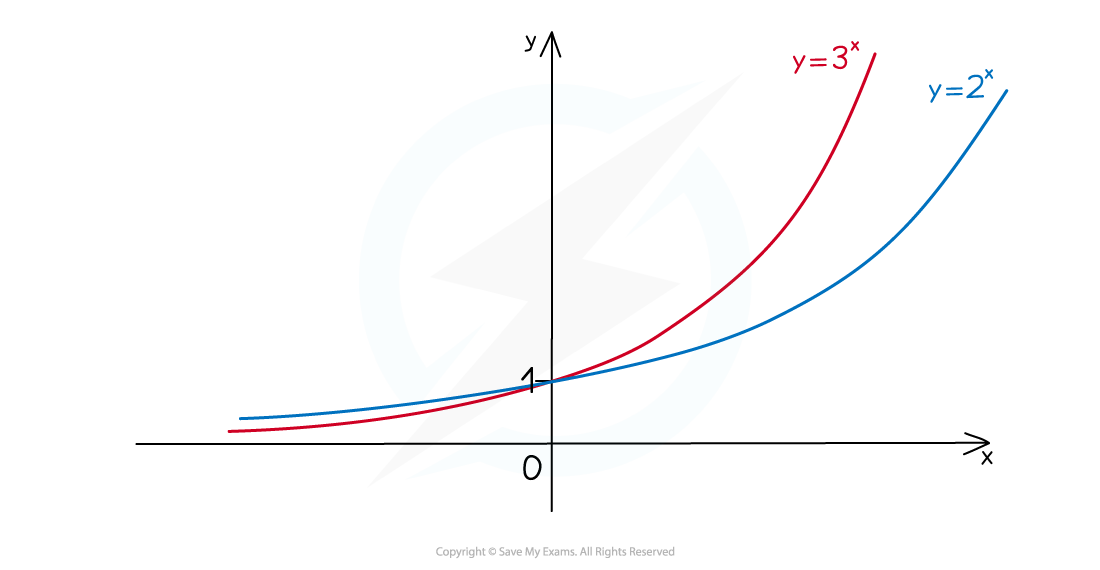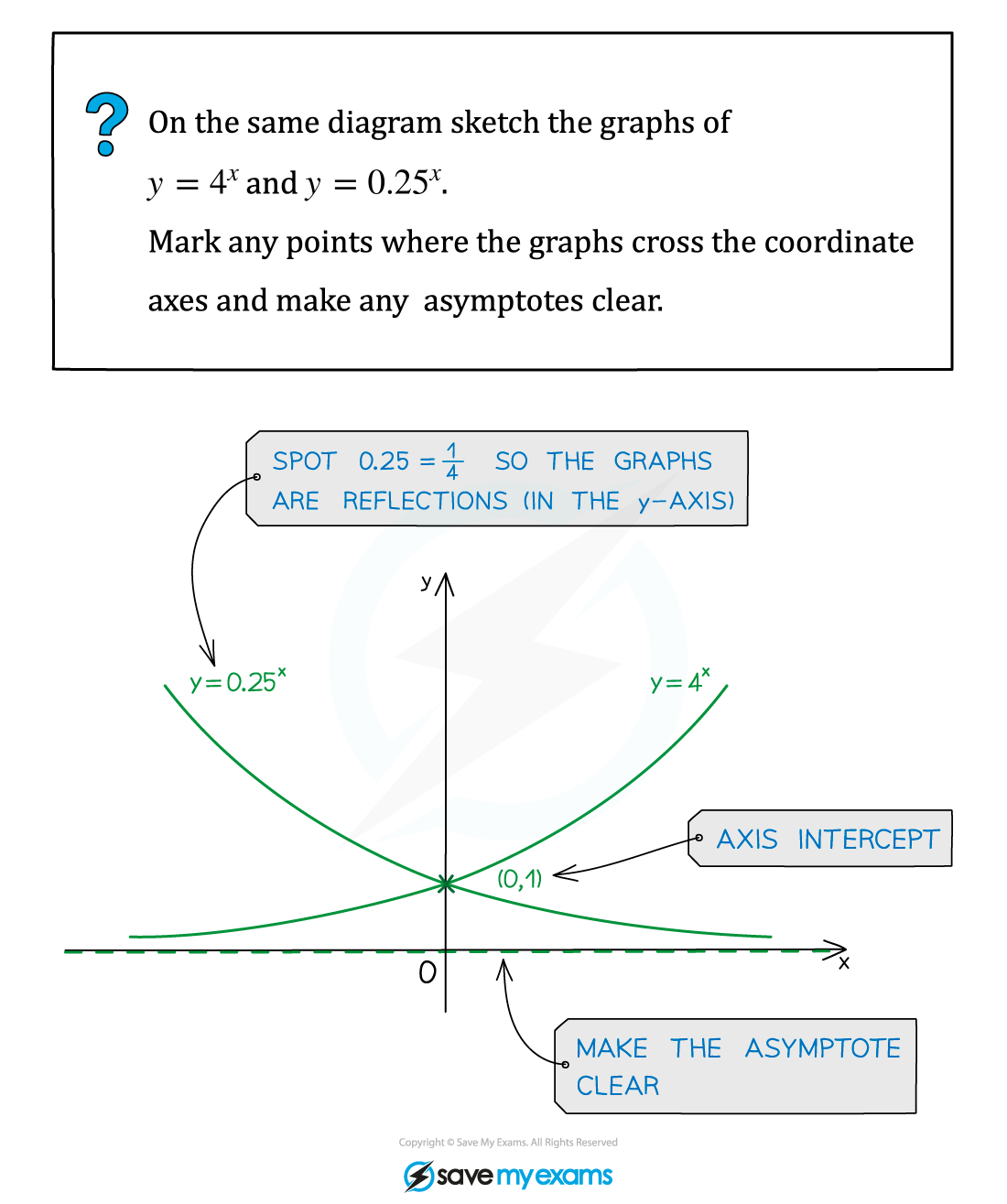# AQA A Level Maths: Pure复习笔记6.1.1 Exponential Functions

### Exponential Functions

#### Exponential functions

• Exponential functions of the form y = ax with a > 0 are considered at A level

#### Exponential graphs

• All graphs of the form y = ax will pass through (0, 1) because a0 = 1
• The x-axis is an asymptote
•#### a > 1

• Where x < 0 the higher value of a is the “lower” graph
• Where x > 0 the higher value of a is the “higher” graph
• a > 1 is exponential growth (see Exponential Growth & Decay)#### 0 < a < 1

• Where x < 0 the higher value of a is the “higher” graph
• Where x > 0 the higher value of a is the “lower” graph
• 0 < a < 1 is exponential decay#### What about when a = 1?

• You may like to think about why a = 1 is not considered... If a = 1, y = 1x = 1 for all values of x

#### Worked Example